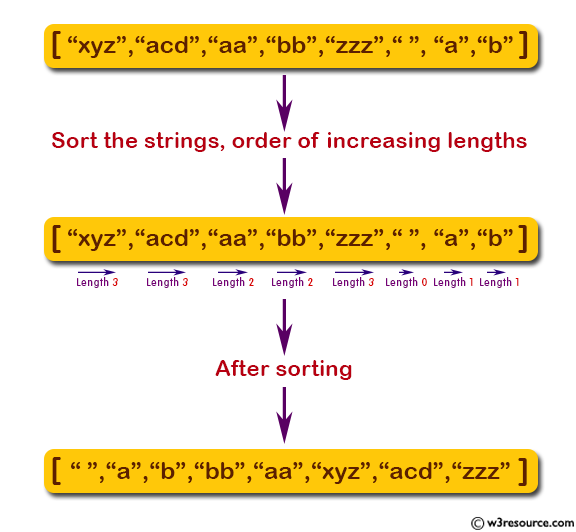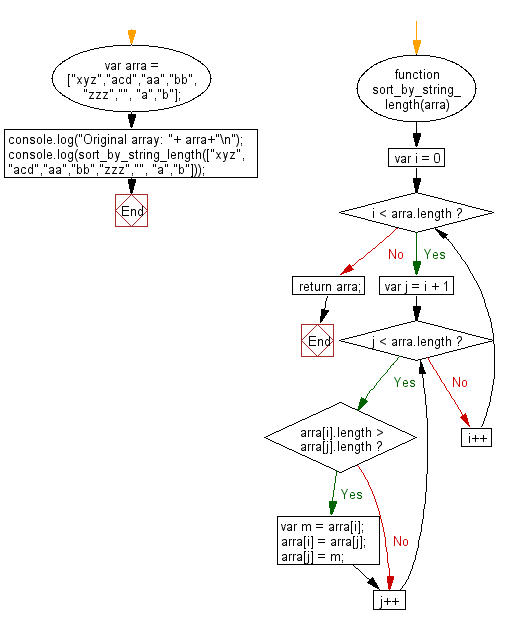# JavaScript: Sort the strings of a given array of strings in the order of increasing lengths

## JavaScript Basic: Exercise-143 with Solution

Write a JavaScript program to sort the strings of a given array of strings in the order of increasing lengths.

Note: Do not change the order if the lengths of two string are same.

Pictorial Presentation:Sample Solution:

HTML Code:

``````<!DOCTYPE html>
<html>
<meta charset="utf-8">
<meta name="viewport" content="width=device-width">
<title>Sort the strings of a given array of strings in the order of increasing lengths.</title>
<body>

</body>
</html>
```
```

JavaScript Code:

``````function sort_by_string_length(arra) {
for (var i = 0; i < arra.length; i++) {
for (var j = i + 1; j < arra.length; j++) {
if (arra[i].length > arra[j].length) {
var m = arra[i];
arra[i] = arra[j];
arra[j] = m;
}
}
}
return arra;
}
var arra = ["xyz","acd","aa","bb","zzz","", "a","b"];
console.log("Original array: "+ arra+"\n");
console.log(sort_by_string_length(["xyz","acd","aa","bb","zzz","", "a","b"]));
``````

Sample Output:

```Original array: xyz,acd,aa,bb,zzz,,a,b

["","a","b","bb","aa","xyz","acd","zzz"]
```

Flowchart:ES6 Version:

``````function sort_by_string_length(arra) {
for (let i = 0; i < arra.length; i++) {
for (let j = i + 1; j < arra.length; j++) {
if (arra[i].length > arra[j].length) {
const m = arra[i];
arra[i] = arra[j];
arra[j] = m;
}
}
}
return arra;
}
var arra = ["xyz","acd","aa","bb","zzz","", "a","b"];
console.log("Original array: ",arra);
console.log(sort_by_string_length(["xyz","acd","aa","bb","zzz","", "a","b"]));
``````

Live Demo:

See the Pen javascript-basic-exercise-143 by w3resource (@w3resource) on CodePen.

Improve this sample solution and post your code through Disqus

What is the difficulty level of this exercise?

Test your Programming skills with w3resource's quiz.

﻿

## JavaScript: Tips of the Day

Checks if a string is an anagram of another string (case-insensitive, ignores spaces, punctuation and special characters)

Example:

```const isAnagram = (str1, str2) => {
const normalize = str =>
str
.toLowerCase()
.replace(/[^a-z0-9]/gi, '')
.split('')
.sort()
.join('');
return normalize(str1) === normalize(str2);
};
console.log(isAnagram('iceman', 'cinema')); // true
```

Output:

```true
```